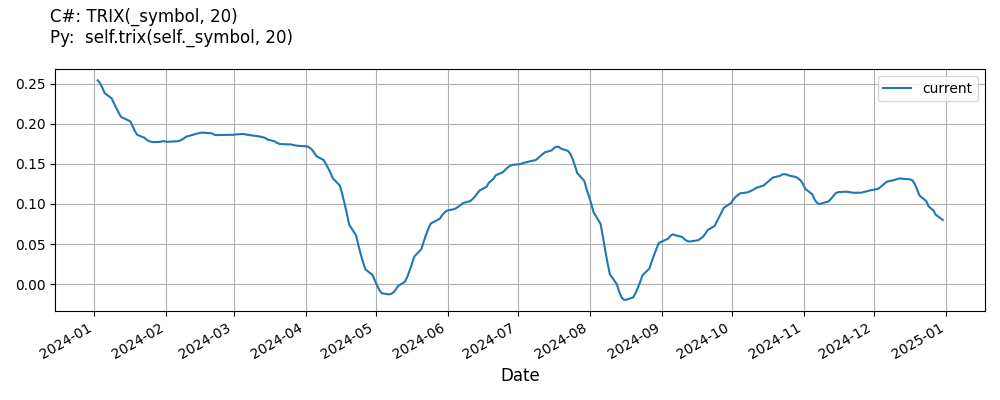# Supported Indicators

## Trix

### Introduction

This indicator computes the TRIX (1-period ROC of a Triple EMA) The TRIX is calculated as explained here: http://stockcharts.com/school/doku.php?id=chart_school:technical_indicators:trix

To view the implementation of this indicator, see the LEAN GitHub repository.

### Using TRIX Indicator

To create an automatic indicators for Trix, call the TRIX helper method from the QCAlgorithm class. The TRIX method creates a Trix object, hooks it up for automatic updates, and returns it so you can used it in your algorithm. In most cases, you should call the helper method in the Initialize method.

public class TrixAlgorithm : QCAlgorithm
{
private Symbol _symbol;
private Trix _trix;

public override void Initialize()
{
_trix = TRIX(_symbol, 20);
}

public override void OnData(Slice data)
{
{
// The current value of _trix is represented by itself (_trix)
// or _trix.Current.Value
Plot("Trix", "trix", _trix);

}
}
}
class TrixAlgorithm(QCAlgorithm):
def Initialize(self) -> None:
self.trix = self.TRIX(self.symbol, 20)

def OnData(self, slice: Slice) -> None:
# The current value of self.trix is represented by self.trix.Current.Value
self.Plot("Trix", "trix", self.trix.Current.Value)



The following reference table describes the TRIX method:

### TRIX()1/1

            Trix QuantConnect.Algorithm.QCAlgorithm.TRIX (
Symbol                           symbol,
Int32                            period,
*Nullable<Resolution>      resolution,
*Func<IBaseData, Decimal>  selector
)


Creates a new Trix indicator.

If you don't provide a resolution, it defaults to the security resolution. If you provide a resolution, it must be greater than or equal to the resolution of the security. For instance, if you subscribe to hourly data for a security, you should update its indicator with data that spans 1 hour or longer.

You can manually create a Trix indicator, so it doesn't automatically update. Manual indicators let you update their values with any data you choose.

Updating your indicator manually enables you to control when the indicator is updated and what data you use to update it. To manually update the indicator, call the Update method with time/number pair, or an IndicatorDataPoint. The indicator will only be ready after you prime it with enough data.

public class TrixAlgorithm : QCAlgorithm
{
private Symbol _symbol;
private Trix _trix;

public override void Initialize()
{
_trix = new Trix(20);
}

public override void OnData(Slice data)
{
if (data.Bars.TryGeValue(_symbol, out var bar))
{
_trix.Update(bar.EndTime, bar.Close);
}

{
// The current value of _trix is represented by itself (_trix)
// or _trix.Current.Value
Plot("Trix", "trix", _trix);

}
}
}
class TrixAlgorithm(QCAlgorithm):
def Initialize(self) -> None:
self.trix = Trix(20)

def OnData(self, slice: Slice) -> None:
bar = slice.Bars.get(self.symbol)
if bar:
self.trix.Update(bar.EndTime, bar.Close)

# The current value of self.trix is represented by self.trix.Current.Value
self.Plot("Trix", "trix", self.trix.Current.Value)



To register a manual indicator for automatic updates with the security data, call the RegisterIndicator method.

public class TrixAlgorithm : QCAlgorithm
{
private Symbol _symbol;
private Trix _trix;

public override void Initialize()
{
_trix = new Trix(20);
RegisterIndicator(_symbol, _trix, Resolution.Daily);
}

public override void OnData(Slice data)
{
{
// The current value of _trix is represented by itself (_trix)
// or _trix.Current.Value
Plot("Trix", "trix", _trix);

}
}
}
class TrixAlgorithm(QCAlgorithm):
def Initialize(self) -> None:
self.trix = Trix(20)
self.RegisterIndicator(self.symbol, self.trix, Resolution.Daily)

def OnData(self, slice: Slice) -> None:
# The current value of self.trix is represented by self.trix.Current.Value
self.Plot("Trix", "trix", self.trix.Current.Value)



The following reference table describes the Trix constructor:

### Trix()1/2

            Trix QuantConnect.Indicators.Trix (
string  name,
int     period
)


Initializes a new instance of the Trix class using the specified name and period.

### Trix()2/2

            Trix QuantConnect.Indicators.Trix (
int  period
)


Initializes a new instance of the Trix class using the specified period.

### Visualization

The following image shows plot values of selected properties of Trix using the plotly library.You can also see our Videos. You can also get in touch with us via Discord.